All kinds of lattices

Posted on 2019-07-23 by Oleg Grenrus

This post contains excerpts from a PDF "post" I have written. It looks way better in PDF.

Partial ordered set, or poset for short is well used example in category theory books. Yet, posets are not too familiar for an average CT-curious Haskeller, yet they are easy to visualise! Let’s do exactly that, and mention elementery bits of category theory. In particular, I’ll scan over the six first chapters of Category Theory by Steve Awodey, repeating related definitions and poset examples.1

Categories

Definition (Awodey 1.1) A category consist of the following data

• Objects: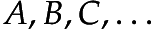• Arrows: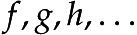• For each arrow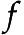, there are given objects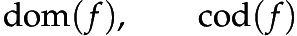called the domain and codomain of. We write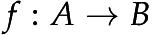to indicate that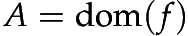and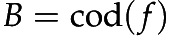.

• Given arrows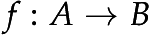and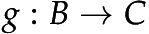, that is, with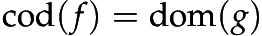there is given an arrow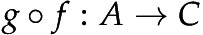called the composite ofand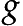.

• For each object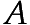, there is given an arrow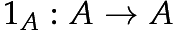called the identity arrow of.

• Associativity: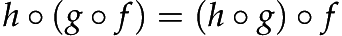for all,,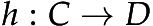.

• Unit: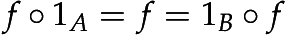for all.

We’ll see how Category type-class is related later, in later section.

Functor

Before proceeding, we’ll answer a question: is there a category with posets as objects? Yes, it’s called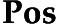! What are the arrows in this category? An arrow from a posetto a poset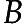is a function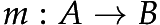that is monotone, in the sense that, for all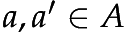,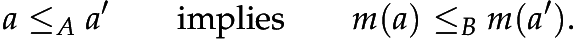We need to know that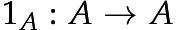is monotone, and also that ifandare monotone, thenis monotone. That holds, check!

Recall, posets are categories, so monotone functions are "mappings" between categories. A "homomorphism2 of categories" is called a functor.

Definition (Awodey 1.2) A functor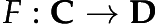between categories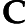and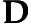is a mapping of objects to objects and arrows to arrows, in such a way that

•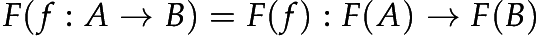,

•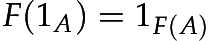,

•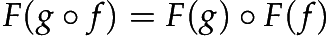.

That is,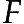preserves domains and codomains, identity arrows, and composition. A functor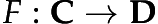thus gives a sort of "picture" – perhaps distorted – ofin.

The  version looks quite different:

class Functor f where
fmap :: (a -> b) -> f a -> f b

There is a mismatch of a notation of category theory, and what can be written as code. In CT notationacts both on objects and arrows. In  f acts on objects, and fmap f acts on arrows. Substituting above, id and . into definition of functor, will also give familiar laws

fmap id       = id
fmap (g . f)  = fmap g . fmap f

However,  Functor-class is only for functors from a pseudo-category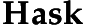to itself, where f, a mapping from types to types is a type-constructor, not arbitrary type family. Functor is a very special case of category theoretical variant.

With small posets, like Bool and M2 we can visualise a monotone function, a functor. Let’s consider a function

f :: Bool -> M2
f True   = M2i
f False  = M2o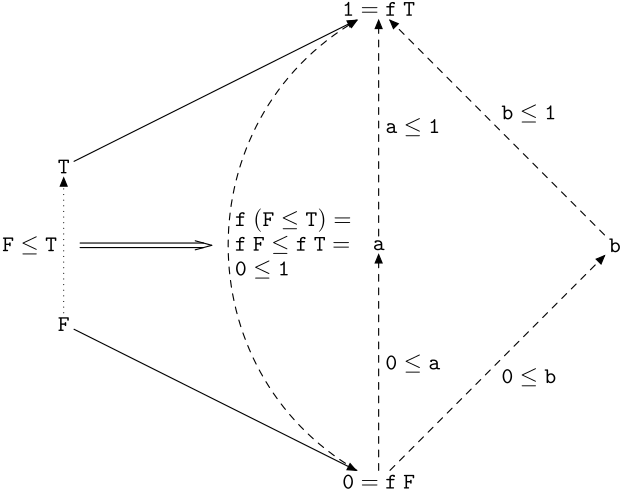Graph of f :: Bool -> M2

The graph of f is on [fig:f-bool-to-m2]. Dotted and dashed lines are arrows in Bool and M2 respectively. We can see on figure, that f indeed gives a picture of Bool in M2.

In  we have only written a mapping of objects, True and False. The mapping of arrows is something we need to check, to be able to claim that f is a functor, and therefore a monotone function. The other way around, there are mappings from Bool to M2 which aren’t monotone, and aren’t functors.

In this section we went backwards. More principal approach would been to first consider functors between poset categories. The monotonicity requirement is implied by first functor requirement. This is a power of category theory. When you look for something, category theory tells you which properties it should have. Once you find something which satisfies the requirements, you know that it’s the right one (up to an isomorphism).

Exponential lattices with M2 are pretty. ZHO -> M2 ([fig:zho2m2]) has nice planar graph. . Yet the final stress test is (ZHO -> ZHO) -> ZHO, or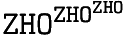is on [fig:big]. A beautiful monster.